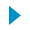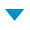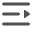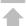•SequoiaDB 简介
•快速入门
•安装部署
•数据库实例
•分布式引擎
•SAC 管控中心
•SequoiaPerf 性能分析工具
•参考手册
•SequoiaDB Shell方法
•连接器
•操作符
•匹配符
•选择符
•函数操作
•更新符
•聚集符
•字段操作符
•SQL语法
•编目表
•数据库快照
•数据库列表
•访问计划的评估过程
•Web服务
•常见问题及解答（FAQ）

# \$multiply

## 语法

`{ <字段名>: { \$multiply: <值> } }`

## 示例

`> db.sample.employee.insert( { "a": 3 } )`
• 作为选择符使用，返回字段 a 乘以 10 的结果

```> db.sample.employee.find( {}, { "a": { "\$multiply": 10 } } )
{
"_id": {
"\$oid": "58256a7b2b4c38286d000020"
},
"a": 30
}
Return 1 row(s).```
• 与匹配符配合使用，匹配字段 a 乘以 10 之积为 30 的记录

```> db.sample.employee.find( { "a": { "\$multiply": 10, "\$et": 30 } } )
{
"_id": {
"\$oid": "58256a7b2b4c38286d000020"
},
"a": 3
}
Return 1 row(s).```收起